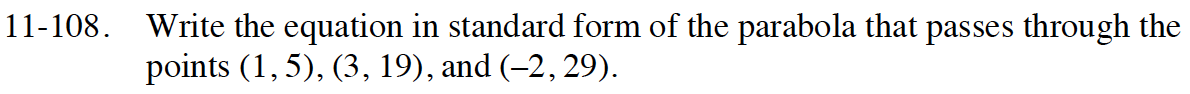Home > INT3 > Chapter 11 > Lesson 11.2.4 > Problem11-108

11-108.

Write the equation in standard form of the parabola that passes through the points (1, 5), (3, 19), and (–2, 29). 11-108 HW eTool (Desmos). Desmos Accessibility Homework Help ✎Substitute the point (1, 5) into y = ax² + bx + c where x = 1 and y = 5.

Repeat the previous step with (3, 19) and (−2, 29) to create two more equations.

You have created a system of three equations with three variables. Solve the system of equations.
Refer to problem 11-100 for help.

a + b + c = 5
9a + 3b + c = 19
4 − 2b + c = 29

(a, b, c) = (3, −5, 7). Substitute for a, b, and c to find the equation of the parabola.

Use the eTool below to graph the answer.
Click the link at right for the full version of the eTool: INT3 11-108 HW eTool